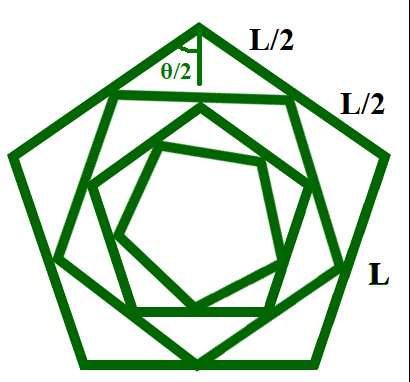# Find the length of Kth N-sided polygon formed by given operations

• Last Updated : 10 May, 2021

Given an integer L, representing the side length of an N-sided regular polygon and integer K, the task is to find the side length of the Kth N-sided regular polygon formed inside the (K – 1)th regular polygon by connecting midpoints of the sides of the (K – 1)th polygon.

Examples:

Input: N = 3, L = 6, K = 2
Output: 3

Input: N = 5, L = 21, K = 7
Output: 5.88796

Approach: The given problem can be solved based on the following observations:• Suppose,  \theta represents the interior angle of the N-sided polygon which is the same for all the polygons formed inside i.e.,• The length of the side of the first polygon formed inside by connecting midpoints of the sides can be calculated using the formula as.
• The length of the side of the Kth polygon formed inside the (K – 1)th polygon and connecting midpoints of the sides of the (K – 1)th polygon isFollow the steps below to solve the problem:

• Find the interior angle of the N-sided regular polygon and store it in a variable say angle in radians.
• Print the side length after calculating the side length of Kth N sided regular polygon by the above-discussed formula.

Below is the implementation of the above approach:

## C++

 // C++ program for the above approach   #include  using namespace std; #define PI 3.14159265   // Function to calculate the interior // angle of a N-sided regular polygon double findInteriorAngle(int n) {     return (n - 2) * PI / n; }   // Function to find the K-th polygon // formed inside the (K - 1)th polygon double calculateSideLength(double L,                            int N, int K) {     // Stores the interior angle     double angle = findInteriorAngle(N);       // Stores the side length of     // K-th regular polygon     double length = L * pow(sin(angle / 2),                             (K - 1));       // Return the length     return length; }   // Driver Code int main() {     double N = 5, L = 21, K = 7;     cout << calculateSideLength(L, N, K);       return 0; }

## Java

 // Java program for the above approach import java.util.*;   class GFG{       static final double PI = 3.14159265;   // Function to calculate the interior // angle of a N-sided regular polygon static double findInteriorAngle(int n) {     return ((n - 2) * PI) / n; }   // Function to find the K-th polygon // formed inside the (K - 1)th polygon static double calculateSideLength(double L,                                   int N, int K) {           // Stores the interior angle     double angle = findInteriorAngle(N);       // Stores the side length of     // K-th regular polygon     double length = L * Math.pow(Math.sin(angle / 2),                                              (K - 1));       // Return the length     return length; }   // Driver Code public static void main(String[] args) {     double L = 21;     int N = 5, K = 7;           System.out.print(calculateSideLength(L, N, K)); } }   // This code is contributed by 29AjayKumar

## Python3

 # Python3 program for the above approach import math PI = 3.14159265   # Function to calculate the interior # angle of a N-sided regular polygon def findInteriorAngle(n):       return (n - 2) * PI / n   # Function to find the K-th polygon # formed inside the (K - 1)th polygon def calculateSideLength(L,                         N, K):       # Stores the interior angle     angle = findInteriorAngle(N)       # Stores the side length of     # K-th regular polygon     length = L * pow(math.sin(angle / 2),                      (K - 1))       # Return the length     return length     # Driver Code if __name__ == "__main__":       N = 5     L = 21     K = 7     print(calculateSideLength(L, N, K))       # This code is contributed by ukasp.

## C#

 // C# program for the above approach using System;   class GFG{       static readonly double PI = 3.14159265;   // Function to calculate the interior // angle of a N-sided regular polygon static double findInteriorAngle(int n) {     return ((n - 2) * PI) / n; }   // Function to find the K-th polygon // formed inside the (K - 1)th polygon static double calculateSideLength(double L,                                   int N, int K) {           // Stores the interior angle     double angle = findInteriorAngle(N);       // Stores the side length of     // K-th regular polygon     double length = L * Math.Pow(Math.Sin(angle / 2),                                              (K - 1));       // Return the length     return length; }   // Driver Code public static void Main(String[] args) {     double L = 21;     int N = 5, K = 7;           Console.Write(calculateSideLength(L, N, K)); } }   // This code is contributed by 29AjayKumar

## Javascript

 

Output:

5.88796

Time Complexity: O(log K)
Auxiliary Space: O(1)

My Personal Notes arrow_drop_up
Recommended Articles
Page :